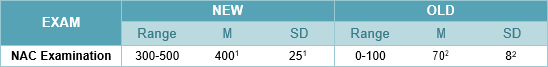# How do I compare the new NAC scores with the old NAC exams?

Need to compare the new exam scores with the old exam scores?

Refer to this chart for the NAC examination:1 Based on candidates in March 2019

2 Based on candidates in March 2013

• New exam score of 475 = three standard deviations above the mean AND SIMILAR PERFORMANCE to an old exam score of 94
• New exam score of 450 = two standard deviations above the mean AND SIMILAR PERFORMANCE to an old exam score of 86
• New exam score of 425 = one standard deviation above the mean AND SIMILAR PERFORMANCE to an old exam score of 78
• New exam score of 400 = mean AND SIMILAR PERFORMANCE to an old exam score of 70
• New exam score of 375 = one SD below the mean AND SIMILAR PERFORMANCE to an old exam score of 62
• New exam score of 350 = two SDs below the mean AND SIMILAR PERFORMANCE to an old exam score of 54